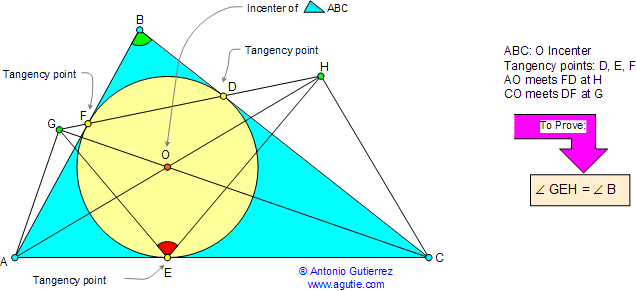< PREVIOUS PROBLEM  |  NEXT PROBLEM > Problem 52. Triangle, angles, incircle. Level: High School, SAT Prep, College In the figure below, a circle of center O inscribed in a triangle ABC is tangent to sides BC, AC, and AB at points D, E, and F, respectively. Lines AO and FD meet at H, and lines CO and DF meet at G. Prove that angle GEH is congruent to angle ABC.Hints: See Geometry Help
 Home | Geometry | Problems | Open Problems | 10 Problems | 51-60 | Wolfram Demonstrations Project | Circle Tangent Line | Email | By Antonio Gutierrez